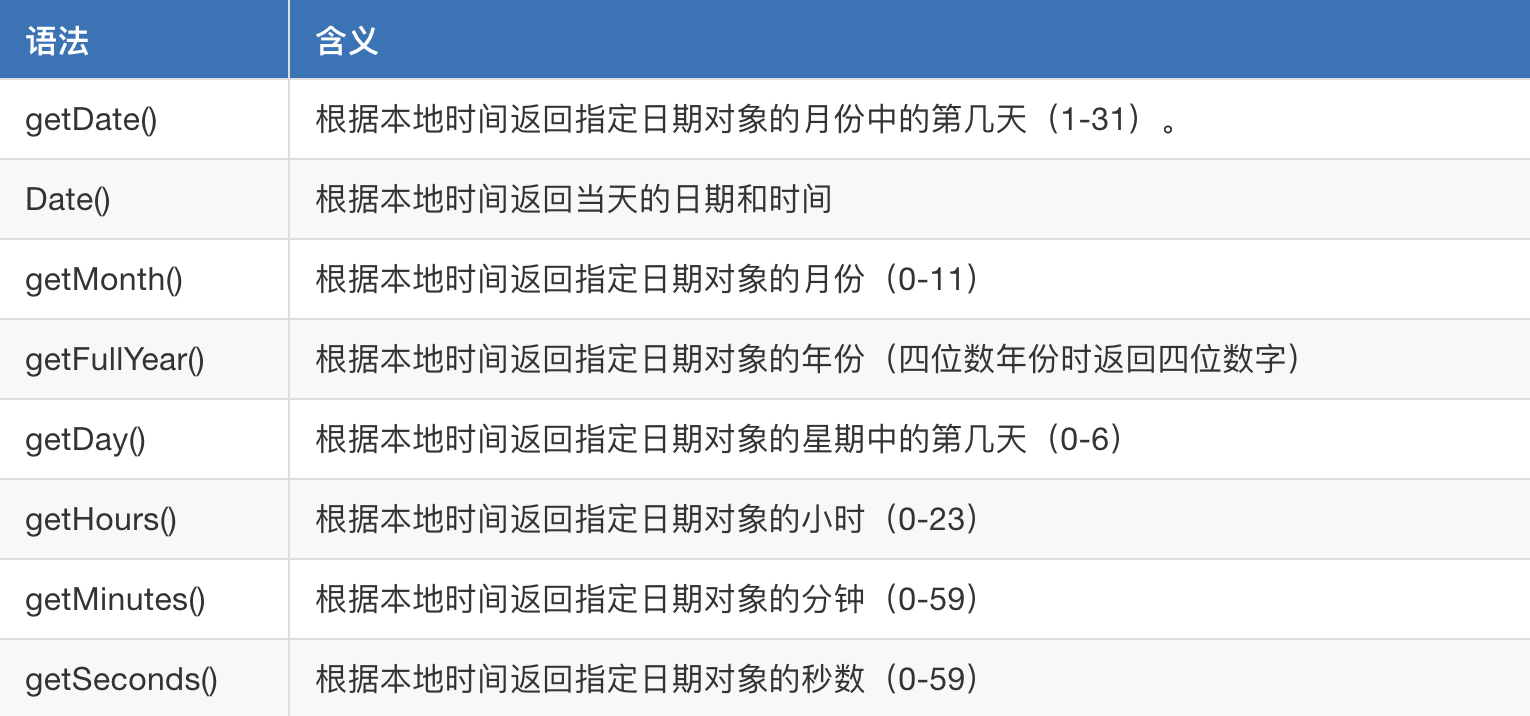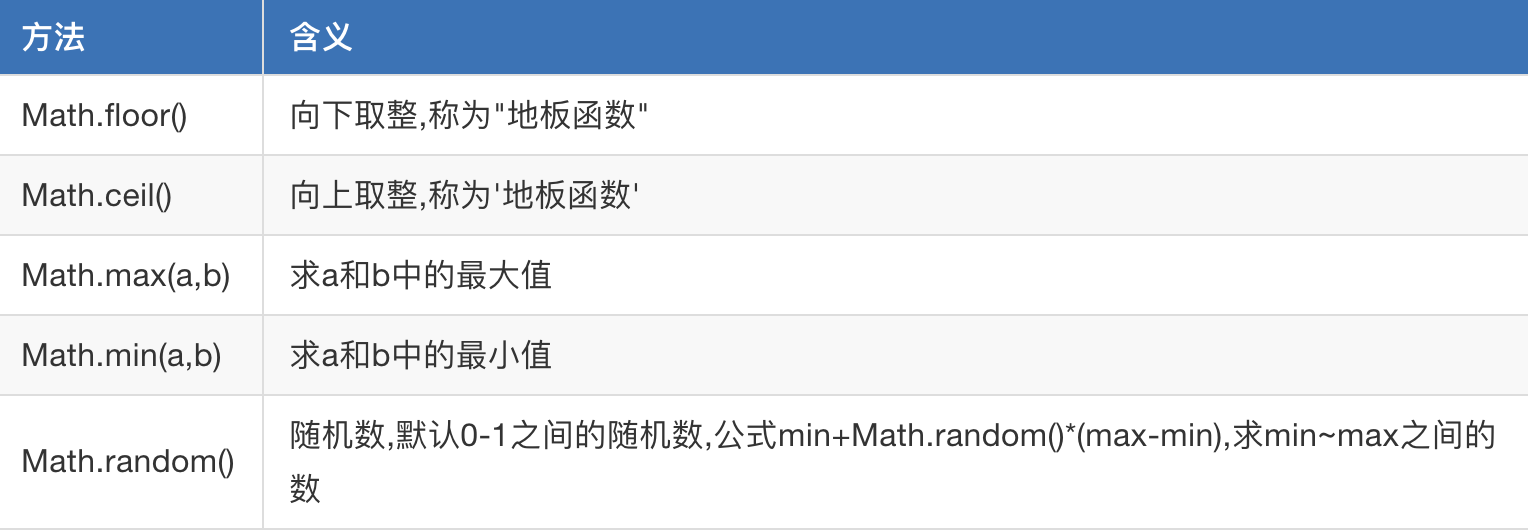# 1. 常用内置对象

## 1.1 数组Array

### 1.1.1 数组的创建方式

var colors = ['red','color','yellow'];

var colors2 = new Array();

### 1.1.2 数组的赋值

var arr = [];
//通过下标进行一一赋值
arr = 123;
arr = '哈哈哈';
arr = '嘿嘿嘿'

### 1.1.3 数组的常用方法

#### (1) concat()：数组的合并

var north = ['北京','山东','天津'];
var south = ['东莞','深圳','上海'];

var newCity = north.concat(south);
console.log(newCity)

#### (2) join()：将数组中的元素使用指定的字符串连接起来，它会形成一个新的字符串

var score = [98,78,76,100,0];
var str = score.join('|');
console.log(str);//98|78|76|100|0

#### (3) toString()：将数组转换成字符串

var score = [98,78,76,100,0];
//toString() 直接转换为字符串  每个元素之间使用逗号隔开

var str = score.toString();
console.log(str);//98,78,76,100,0

#### (4) slice(start,end)：返回数组的一段，左闭右开

var arr = ['张三','李四','王文','赵六'];
var newArr  = arr.slice(1,3);
console.log(newArr);//["李四", "王文"]

#### (5) pop()：删除数组的最后一个元素并返回删除的元素

var arr = ['张三','李四','王文','赵六'];
var item = arr.pop();
console.log(arr);//["张三", "李四"，"王文"]
console.log(item);//赵六

#### (6) push()：向数组末尾添加一个元素或多个元素，并返回新的长度

var arr = ['张三','李四','王文','赵六'];
var newLength= arr.push('小马哥');//可以添加多个，以逗号隔开
console.log(newLength);//5
console.log(arr);//["张三", "李四"，"王文"，"赵六"，"小马哥"]

#### (7) reverse()：翻转数组

var names = ['alex','xiaoma','tanhuang','angle'];

//4.反转数组
names.reverse();
console.log(names);

#### (8) sort()：对数组排序

var names = ['alex','xiaoma','tanhuang','abngel'];
names.sort();
console.log(names)；// ["alex", "angle", "tanhuang", "xiaoma"]

#### (9) isArray()：判断是否为数组

布尔类型值 = Array.isArray(被检测的值) ;

#### (10) shift()：删除并返回数组的第一个元素

var arr = ['张三','李四','王文','赵六'];
var a = arr.shift();
console.log(a); //张三
console.log(arr); //['李四','王文','赵六']

#### (11) unshift()：向数组的开头添加一个或更多元素，并返回新的长度

var arr = ['张三','李四','王文','赵六'];
var a = arr.unshift('王五');
console.log(a);  //5
console.log(arr);  //['王五','张三','李四','王文','赵六']

## 1.2 字符串String的常用方法

#### (1) chartAt()：返回指定索引的位置的字符

var str = 'alex';
var charset = str.charAt(1);
console.log(charset);//l

#### (2) concat()：返回字符串值，表示两个或多个字符串的拼接

var str1 = 'al';
var str2  = 'ex';
console.log(str1.concat(str2,str2));//alexex

#### (3) replace(a,b)：将字符串a替换成字符串b

var a = '1234567755';
var newStr = a.replace("4567","****");
console.log(newStr);//123****755

#### (4) indexof()：查找字符的下标，如果找到返回字符串的下标，找不到则返回-1 。跟seach()方法用法一样

var str = 'alex';
console.log(str.indexOf('e'));//2
console.log(str.indexOf('p'));//-1

#### (5) slice(start，end)：提取一个字符串的一部分，并返回一新的字符串。左闭右开 分割字符串

var str = '小马哥';
console.log(str.slice(1,2));//马

#### (6) split('a',1)：以字符串a分割字符串，并返回新的数组。如果第二个参数没写，表示返回整个数组，如果定义了个数，则返回数组的最大长度

var  str =  '我的天呢,a是嘛,你在说什么呢?a哈哈哈';
console.log(str.split('a'));//["我的天呢,", "是嘛,你在说什么呢?", "哈哈哈"]

#### (7) substr(start,length)：返回一个字符串中从指定位置开始到指定字符数的字符

var  str =  '我的天呢,a是嘛,你在说什么呢?a哈哈哈';
console.log(str.substr(0,4));//我的天呢

#### (8) toLowerCase()：转小写

var str = 'XIAOMAGE';
console.log(str.toLowerCase())；//xiaomage

#### (9) toUpperCase()：转大写

var str = 'xiaomage';
console.log(str.toUpperCase());

#### (11) trim()：去除字符串两边的空白

var str = '   xhh   ';
console.log(str);
console.log(str.trim());

## 1.3 Date日期对象

//创建了一个date对象
var myDate = new Date();//创建日期对象
var myDate=new Date();

//获取一个月中的某一天
console.log(myDate.getDate());

//返回本地时间
console.log(myDate().toLocalString());//2018/5/27 下午10:36:23

## 1.4 Math内置对象#### (1) Math.ceil() 向上取整

var x = 1.234;
//天花板函数  表示大于等于 x，并且与它最接近的整数是2
var a = Math.ceil(x);
console.log(a);//2

#### (2) Math.floor()：向下取整

var x = 1.234;
// 小于等于 x，并且与它最接近的整数 1
var b = Math.floor(x);
console.log(b);//1

#### (3) 求两个数的最大值和最小值

//求 两个数的最大值 最小值
console.log(Math.max(2,5));//5
console.log(Math.min(2,5));//2

#### (4) 随机数：Math.random()

var ran = Math.random();
console.log(ran); //[0,1)

# 2. 函数

console.log("hello world");
sayHello();     //调用函数
//定义函数：
function sayHello(){
console.log("hello");
console.log("hello world");
}

## 2.1 函数的定义

 function 函数名字(){

}

function：是一个关键字。中文是“函数”、“功能”。

## 2.2 函数的调用

 函数名字();

### 2.2.1 函数的参数：形参和实参

sum(3,4);
sum("3",4);
sum("Hello","World");

//函数：求和
function sum(a, b) {
console.log(a + b);
}

### 2.2.2 函数的返回值

console.log(sum(3, 4));

//函数：求和
function sum(a, b) {
return a + b;
}
posted @ 2019-11-18 14:05  爱编程的小灰灰  阅读(126)  评论(0编辑  收藏